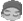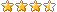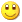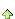共有9972人关注过本帖树形打印复制链接

# 主题：[推荐]Java授课视频第六课：面向对象――封装之一1楼 博客 | 信息 | 搜索 | 邮箱 | 主页 | UC加好友发短信管理员

[推荐]Java授课视频第六课：面向对象――封装之一Post By：2011/3/25 12:45:14 [只看该作者]

 媒体文件信息文件来源：http://www.njcie.com/JavaSE/files/第六课：面向对象封装之一.wmv您可以点击控件上的播放按钮在线播放。注意，播放此媒体文件存在一些风险。附加说明：动网论坛系统禁止了该文件的自动播放功能。由于该用户没有发表自动播放多媒体文件的权限或者该版面被设置成不支持多媒体播放。 [此贴子已经被作者于2011-03-25 12:47:21编辑过]2楼 博客 | 信息 | 搜索 | 邮箱 | 主页 | UC加好友发短信管理员

 //时钟类 class Clock {        private int hour;        private int minute;        private int second;         public Clock() {         }         public Clock(int h, int m, int s) {                setTime(h, m, s);        }         public int getHour() {                return hour;        }         public void setHour(int hour) {                if (hour >= 0 && hour <= 23)                        this.hour = hour;                else if (hour > 0)                        this.hour = hour % 24;                else                        this.hour = 0;        }         public int getMinute() {                return minute;        }         public void setMinute(int minute) {                if (minute >= 0 && minute <= 59)                        this.minute = minute;                else                        this.minute = minute % 60;        }         public int getSecond() {                return second;        }         public void setSecond(int second) {                if (second >= 0 && second <= 59)                        this.second = second;                else                        this.second = second % 60;        }         public void setTime(int h, int m, int s) {                setHour(h);                setMinute(m);                setSecond(s);        }         public String toString() {                java.text.DecimalFormat df = new java.text.DecimalFormat("00");                return df.format(hour) + "小时" + df.format(minute) + "分钟"                                + df.format(second) + "秒";        } } public class Exec {        public static void main(String[] args) {                Clock beijing = new Clock(-2, 23, 45);                System.out.println(beijing);         }}3楼 博客 | 信息 | 搜索 | 邮箱 | 主页 | UC加好友发短信管理员

 //分数类 class FS {        private int fz=1;        private int fm=1;         public FS() {         }         public FS(int fz, int fm) {                setFS(fz, fm);        }         public int getFz() {                return fz;        }         public void setFz(int fz) {                this.fz = fz;        }         public int getFm() {                return fm;        }         public void setFm(int fm) {                if (fm != 0)                        this.fm = fm;                else                        fm = 1;        }         public void setFS(int fz, int fm) {                setFz(fz);                setFm(fm);        }         public String toString() {                return fz + "/" + fm;        } } public class Exec {        public static void main(String[] args) {                FS fs = new FS();                fs.setFz(2);                fs.setFm(3);                System.out.println(fs);        }}加好友发短信管理员